A projectile can have the same range R for two angles of projection. If t1 and t2 be the time of flights in the two cases, then the product of the two time of flights is proportional to?

Kavita Singh IIT Physics 10 Aug, 2018 111 views

A ball is thrown from a point with a speed v0 at an angle of projection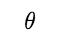. From the same point and at the same instant a person starts running with a constant speed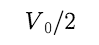to catch the ball. Will the person be able to catch the ball? If yes, what should be the angle of projection?

Anonymous User IIT Physics 10 Aug, 2018 224 views

A projectile can have the same range R for two angles of projection. If T1 and T2 be the time of flights in the two cases, then the product of the two time of flights is directly proportional to?

Anonymous User IIT Physics 10 Aug, 2018 3452 views

## The co­ordinates of a moving particle at any time t are given byThe co­ordinates of a moving particle at any time t are given by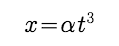and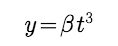. The speed of the particle at time t is given by

Anonymous User IIT Physics 10 Aug, 2018 88 views

A boy playing on the roof of a 10 m high building throws a ball with a speed of 10 m/s at an angle of 30° with the horizontal. How far from the throwing point will the ball be at the height of 10 m from the ground ?

Anonymous User IIT Physics 10 Aug, 2018 821 views

Two forces are such that the sum of their magnitudes is 18 N and their resultant is 12 N which is perpendicular to the smaller force. Then the magnitudes of the forces are?

Anonymous User IIT Physics 10 Aug, 2018 78 views

A particle located at x = 0 at time t = 0, starts moving along the positive x­-direction with a velocity v that varies as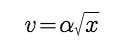.The displacement of the particle varies with time as

Anonymous User IIT Physics 09 Aug, 2018 3063 views